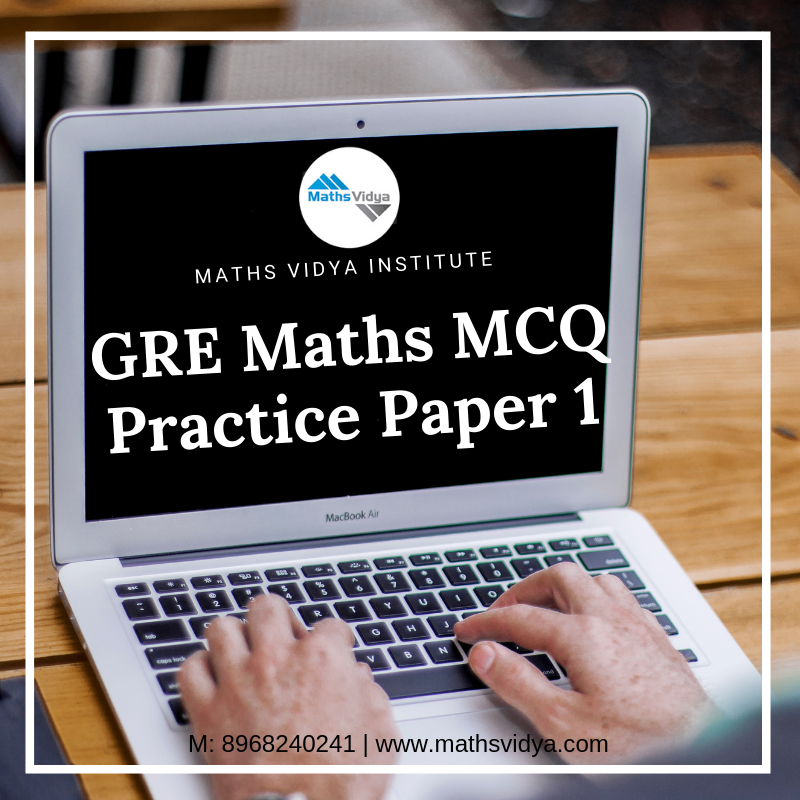# GRE Quantitative Maths Practice MCQ Test 1The Maths Vidya GRE Quantitative Reasoning practice test is a list of multiple-choice questions, which helps you to know where you stand and how much practice you require to get good score in GRE. Maths Vidya is the best institute in Chandigarh offers excellent GRE Maths Coaching.

Question 1:
y = x2 – 1
y = 8

 Column A Column B y/5 x

Question 2:

p is a real number and p > 1

 Column A Column B 1/p 1/2 + 1/5

Question 3:

If the ratio students to teachers on a committee of 21 members is 8 to 6, how many members of the committee are students?

Question 4:

If a, b and c are odd integers, which of the following expressions must be an even integer?

Question 5:

The figure below shows two concentric circles each with center O. The radius of C1 is r1, the radius of C2 is r2, and r1 = (√2)·r2Column A Column B Area of circle C2 Shaded area

Question 6:

Find the solutions of the x2 + 3x – 18 = 0 equation.

Question 7:

The height h of the trapezoid ABCD is equal to the average of its two parallel sides.Column A Column B Area of ABCD h2

Question 8:

In the x,y coordinate system, line l passes through the point P(2, 1) and is perpendicular to line y = x + 1.

 Column A Column B y intercept of line l x intercept of line l

Question 9:

In which of the following years was the difference between the revenues of company A and company B the lowest?Question 10:

What is the approximate percentage of all employees of Company A that are operators?Source URL: http://www.free-test-online.com/gre-general-test/gre-math-test-1